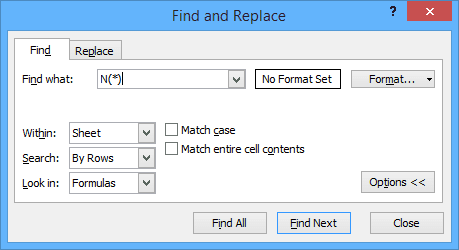## How to Insert Notes in Formulas using a Function in Excel

by Avantix Learning Team | Updated November 24, 2019

Applies to: Microsoft® Excel® 2010, 2013, 2016, 2019 and 365 (Windows)

In addition to adding comments in Excel, there is a relatively obscure function called the N function that you can use to enter notes directly within a formula. The N function converts a value to a number and has been around for a long while so you can use it in older versions of Excel as well.

### Syntax

The N function has the following syntax:

=N(argument)

### Using the N function to enter notes

You can use the N function to enter notes directly in a formula. Simply enter +N(“whatever note you want”) at the end of the formula. The text of the note must appear in double quotes (“”) and returns a value of 0 so it does not affect the calculation.

For example:

=COUNTA(A1:A900)+N(“This sample data set extracted from United Global data 2014”)

### Returned values

The N function returns values as follows:

• If the value is or refers to a number, the N function returns the number so in the formula =N(A1) where A1 contains 400, the returned value would be 400.
• If the value is or refers to a date, the function returns the serial number of the date so in the formula =N(A1) where A1 contains 1/1/2014, the returned value would be 41640 or the number of days from the first date in the system.
• If the value is or refers to TRUE, the N function returns 1.
• If the value is or refers to FALSE, the N function returns 0.
• If the value is or refers to an error value the N function returns the error value.
• If the value is or refers to anything else (like a note in quotes) the N function returns 0.

### Finding formulas with notes

You can find formulas with notes entered using the N function with the Find command:

1. Click the Home tab in the Ribbon.
2. Click Find and Select in the Editing group.
3. Click Find. Alternatively, you can press Ctrl + F. The Find dialog appears.
4. In the Find dialog, enter N( or N(*) in the Find what area.
5. Choose to search within the sheet or workbook and to look in formulas.
6. Click Find. Continue clicking Find for each instance of a note using the N function. You can also click Find All for a listing of all instances.You don’t normally use the N function in a formula to convert values to numbers because Excel automatically converts values. The N function is provided for compatibility with other spreadsheet programs but also provides an interesting way to enter notes in Excel.

#### Recommended Microsoft Excel courses

Microsoft Excel: Data Analysis with Functions, Dashboards and What-If Analysis Tools

Microsoft Excel:  Visual Basic for Applications (VBA) | Introduction

Microsoft Excel: Introduction to Power Pivot and Power Query Business Intelligence Tools

MORE COURSES >

## How to Delete Blank Rows in Excel (Great Strategies, Tricks and Shortcuts for All Versions)

You can delete blank rows in Microsoft Excel worksheets manually or you can use several tricks to delete multiple blank rows quickly. First, it’s essential that Excel users know how  to select rows quickly using shortcuts or by using Excel commands such as Go to Special, Filter and Sort.

Microsoft, the Microsoft logo, Microsoft Office and related Microsoft applications and logos are registered trademarks of Microsoft Corporation in Canada, US and other countries. All other trademarks are the property of the registered owners.

Avantix Learning | 1 Yonge Street, Suite 1801 (Toronto Star Building), Toronto, Ontario, Canada M5E 1W7 | info@avantixlearning.ca

SummaryArticle Name
Hiding Notes in Microsoft Excel (Writing Notes within a Formula)
Description
In addition to adding comments in Excel, there is a relatively obscure function called the N function that you can use to enter notes directly within a formula. The N function converts a value to a number and has been around for a long while so you can use it in older versions of Excel as well.
Author
Publisher Name
Avantix Learning Inc.